StatLect

# The plug-in principle

The plug-in principle is a technique used in probability theory and statistics to approximately compute or to estimate a feature of a probability distribution (e.g., the expected value, the variance, a quantile) that cannot be computed exactly. It is widely used in the theories of Monte Carlo simulation and bootstrapping.

Roughly speaking, the plug-in principle says that a feature of a given distribution can be approximated by the same feature of the empirical distribution of a sample of observations drawn from the given distribution. The feature of the empirical distribution is called a plug-in estimate of the feature of the given distribution. For example, a quantile of a given distribution can be approximated by the analogous quantile of the empirical distribution of a sample of draws from the given distribution.## Definition

The following is a formal definition of plug-in estimate.

Definition Letbe a set of distribution functions. Letbe a mapping. Let. Let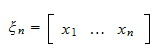be a sample of realizations ofrandom variables, ...,all having distribution function. Letbe the empirical distribution function of the sample. If, then the quantityis called a plug-in estimate of.

The next section will provide an informal discussion of the conditions under whichconverges toas the sample sizeincreases. Before doing that, we will provide some examples to clarify the meaning of the mapping. The next example shows how the plug-in principle can be used to approximate expected values.

Example Suppose we need to compute the expected valuewhereis a random variable andis a function. Ifis the distribution function of, then the expected value can be written as a Riemann-Stieltjes integral (see the lecture entitled Expected value) as follows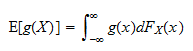We can define a mappingsuch that, for any distribution function, we have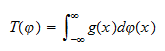Thus, the expected value we need to compute is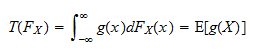Now, if we have a sample ofdraws, ...,from the distribution, their empirical distribution functionis the distribution function of a discrete random variable that can take any of the values, ...,with probability. As a consequence, the plug-in estimate ofis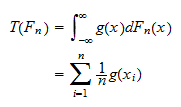The next example shows how the plug-in principle can be used to approximate quantiles.

Example Suppose that we need to compute the-quantileof a random variablehaving distribution function, and suppose that we are not able to compute it by using the definition of-quantile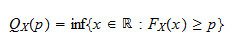We can define a mappingsuch that, for any distribution function, we have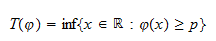Thus, the quantile we need to compute is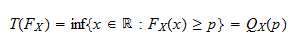If we have a sample ofdraws, ...,from the distribution, and we denote bytheir empirical distribution function, then the plug-in estimate ofis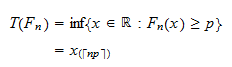whereis the ceiling of, that is, the smallest integer not less than, andis the-th order statistic of the sample, that is, the-th smaller observation in the sample.

## Asymptotic properties

We will not go into the details of the asymptotic properties of plug-in estimators because this would require a level of mathematical sophistication far beyond the level required on average in these lecture notes. However, we will discuss the main issues related to their convergence and provide some intuition.

First of all, one may wonder whether the plug-in estimateconverges in some sense toas the sample sizeincreases. As we have seen in the lecture entitled Empirical distribution, the Glivenko-Cantelli theorem and its generalizations provide sets of conditions under which the empirical distributionconverges to. Ifandwere finite-dimensional vectors, then one could apply the Continuous Mapping theorem and say that ifis continuous, thenconverges to. Unfortunately,andare not finite-dimensional because they are functions defined on(which is uncountable), and, as a consequence, it is not possible to apply the Continuous Mapping Theorem. However, there are several theorems, analogous to the Continuous Mapping theorem, that can be applied in the case of plug-in estimators: if the mappingis continuous in some sense (or differentiable), thenconverges to. The continuity conditions required in these theorems are often complicated and difficult to check in practical cases. We refer the interested reader to van der Vaart (2000) for more details. Rest assured, however, that the most commonly used mappings(e.g., mean, variance, moments and cross-moments, quantiles) satisfy the required continuity conditions.

Furthermore, it is also possible to prove that, under certain conditions, a version of the Central Limit Theorem applies to the plug-in estimate, that is, the quantityconverges in distribution to a normal random variable. Ifandwere finite-dimensional vectors, then one could require thatbe differentiable and apply the Delta Method to prove the asymptotic normality of the above quantity. But sinceandare infinite dimensional, a more general technique, called Functional Delta Method, needs to be employed (which utilizes a notion of differentiability forthat is called Hadamard differentiability). Again, we refer the interested reader to van der Vaart (2000) for more details.

## References

van der Vaart, A. W. (2000) Asymptotic statistics, Cambridge University Press.

The book

Most of the learning materials found on this website are now available in a traditional textbook format.

Glossary entries
Share